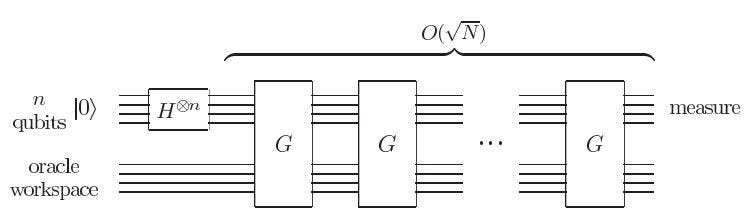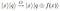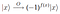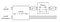# Using Grover’s Algorithm to Solve Cryptarithms Part 1

The number of potential applications for quantum computing has exploded in recent years. One of the algorithms that paved the way for the exploration being done today is the Grover’s Search Algorithm developed by Lov Grover in 1996.

The algorithm provides a quadratic speedup over its corresponding classical implementation. Specifically, Grover’s Algorithm is able to find a particular input that produces a particular output for some blackbox function in O(√N) time. A classical algorithm hoping to accomplish the same feat would have a worst case time performance of O(N) since the worst case would require every item in the set of inputs to be evaluated prior to finding the desired output. The nature of quantum algorithms is that they are inherently probabilistic; Grover’s Algorithm is no different in that it does not provide a single answer with 100% certainty.

The following is a brief overview of the Grover Algorithm closely following Section 6.1.2 of “Quantum Computation and Quantum Information” by Nielsen and Chuang. A more application based overview is available in the GroversAlgorithm Kata on GitHub.

The first step is to encode the input set as orthogonal quantum states. Next, the Hadamard gate is used to generate an equal superposition of these input states. A construct known as the Grover iteration can then be applied to perform a process known as amplitude amplification. This can be seen schematically in the figure below.Schematic of general Grover’s Search Algorithm Circuit with H denoting the Hadamard Gate and G denoting a single Grover Iteration. (Section 6.1.2 of “Quantum Computation and Quantum Information” by Nielsen and Chuang)

To further explain the contents of a Grover iteration, we introduce a concept known as an oracle. This oracle is the “blackbox function” mentioned earlier and is used to verify the correctness of a given input value. The oracle is a unitary operator, denoted O, given by the following equation where |x> represents an input state, |q> is a qubit in the oracle workspace, and the ⊕ symbol represents addition modulo 2:Equation describing the unitary oracle operator. (Section 6.1.2 of “Quantum Computation and Quantum Information” by Nielsen and Chuang)

The nature of the ⊕ operator is such that if f(x) = 1, the qubit |q> is flipped, while if f(x) = 0, |q> remains unchanged. If we now were to represent |q> in a superposition state such that flipping |q> could be fully described by a change in sign, we are able to simplify the oracle behavior to the following:Simplified equation describing the unitary oracle operator. (Section 6.1.2 of “Quantum Computation and Quantum Information” by Nielsen and Chuang)

This element allows us to mark the input state corresponding to our desired output state with a negative amplitude. In the application of Grover’s Algorithm to cryptarithmic problems, developing an oracle that captures this behavior is a major challenge.

The next and final concept to introduce is the conditional phase shift. This applies a negative amplitude to every state unless the state is |0>. Applying a Hadamard gate before and after this conditional phase shift causes the probability of measuring the input state that caused the oracle function to output a value of 1 to increase. This is known as amplitude amplification. We note that this step of the Grover’s Algorithm is referred to in literature as a Grover diffusion operator

A summary of the oracle and conditional phase shift step can be seen in the schematic of a single Grover iteration below:Schematic for a single Grover Iteration, G. (Section 6.1.2 of “Quantum Computation and Quantum Information” by Nielsen and Chuang)

Having developed some background on the inner workings of Grover’s Algorithm, we can begin to describe how it can be utilized to solve cryptarithms. Cryptarithms are puzzles where a series of letters are used to represent digits in an addition problem. The famous SEND + MORE = MONEY cryptarithm is seen below:

To solve the puzzle, we must find numbers corresponding to each letter that satisfies the above expression as seen in the following figure.

The computational requirements for solving such a problem closely align with constraint satisfaction problems. A rather famous constraint satisfaction problem is graph coloring. Given a set of colors, graph coloring problems require the distribution of these colors onto a graph such that no adjacent nodes contain the same color. The GraphColouring Kata on GitHub illustrates how Grover’s Algorithm can be applied to such problems.

Due to the fact that the Grover's Algorithm is generic, only the oracle step needs to be modified to accommodate the solving of cryptarithms. We use an approach developed by Dr. Marek Perkowski to develop the oracle design of a smaller cryptarithmic problem which can be simulated:

Each letter can take a value from 0–9 which we have chosen to encode in base 4 with the use of 4 qubits. The use of base 4 simplifies the addition behavior required later.

The first restriction is that none of the distinct letters can equal one another: M ≠ O, M ≠ C, M ≠W, C≠O, etc. This is known as an equality oracle and is common between cryptarithmic and graph coloring problems.

The next restriction involves the sums along each column. We begin by defining 2 carryover variables C1, and C2 which can take the value 1 or 0 and are represented by a single qubit each. Looking at the columns of the above puzzle, we can use these carryover variables to describe restrictions per column.

The encoding described by Dr. Marek Perkowski goes on to describe the use of quantum full adders and quantum half adders to satisfy the constraints posed by the above equations. I will be implementing this encoding on Q# and will post a second blog post detailing how this encoding is applied for cryptarithmic problems involving the same number of letters as the simplified example. The second blog post will also detail my procedure for adding a Kata titled SolveCryptarithmsWithGrover onto the QuantumKatas repository.